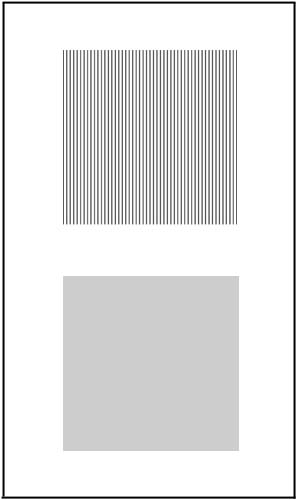# Resolving Power of the EyeThe figure shows two patterns, one made of vertical lines and one which is simply gray. Download a postscript file, resolve.ps and print it on a laser printer. You may need to adjust the darkness of the gray pattern, since different printers make it look different. When you stand 5 meters or more from the figure, the two patterns should look identical with the same shade of gray. To change the darkness of the gray, edit the file, resolve.ps, with a text editor like notepad. On the 10th line, you will find a line, "0.900000 setgray". You can adjust the darkness of the gray pattern by changing the number, 0.9. Decrease it to make the gray darker (0 is black), and increase it to make the gray lighter (1 is white).

In the printed figure, the lines are separated by 2 mm. Viewed at a distance, the two patterns look identical, but as you approach them, there is a point at which you can barely resolve the lines and tell the difference between the two images. From this distance L, you can calculate the angular resolution of your eyes:

angular resolution = (2 mm)/L (in radians).

Classroom demonstration: Hold up the figure and ask who can see the lines in one of the patterns. Usually no one beyond 4 meters will raise their hands. (This works best in a classroom which is 8 meters or more deep.) Using the above equation, L = 4 m corresponds to an angular resolution of 0.03 degrees.

The diffraction limit of the eye can be calculated using Rayleigh's criterion:

angular resolution = (1.22)(lambda)/D,

where lambda is the wavelength of light (on the average, about 550 nm) and D is the diameter of the eye's pupil, which is about 5 mm indoors. This calculation results in an angular resolution of 0.008 degrees. If your eyes could resolve images at the diffraction limit, you could resolve the lines in the printed pattern at a distance of 15 m!